﻿ Price Equal To Value Upon Quantity Equation Written On Chalkboard With Illustrations Stock Illustration - Illustration of mathematical, distance: 165003749

# price equal to value upon quantity equation written on chalkboard with illustrations.

Royalty-Free Illustration## price equal to value upon quantity equation written on chalkboard with illustrations

More similar stock illustrations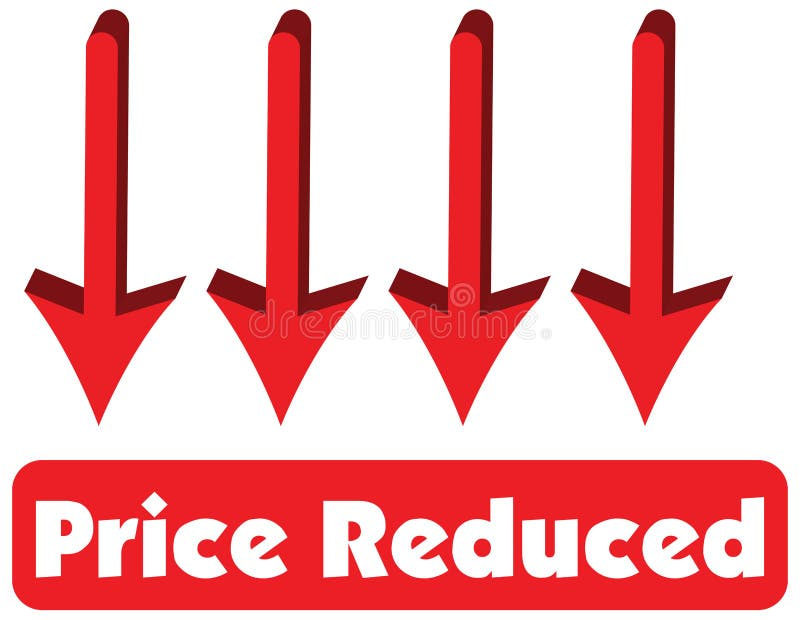Price Reduced concept of red arrow pointing down is equal to price reduced.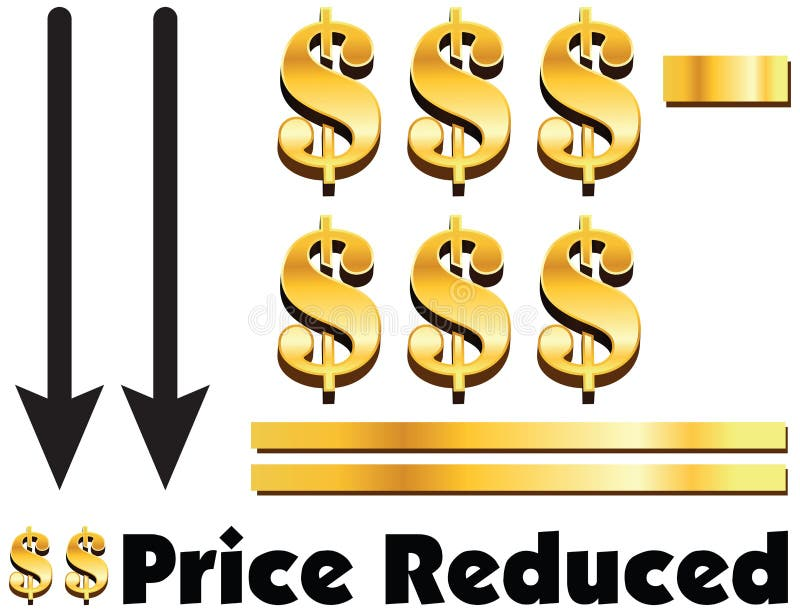Price Reduced concept of dollar minus dollar is equal to price r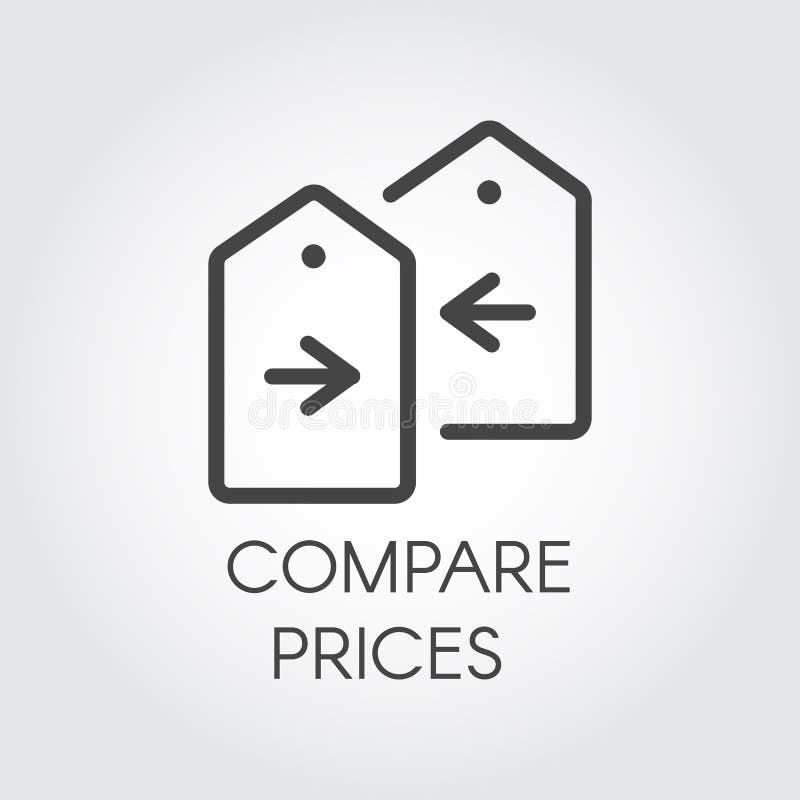Compare prices icon drawing in line design. Financial comparison outline pictogram. Price-tag with arrow label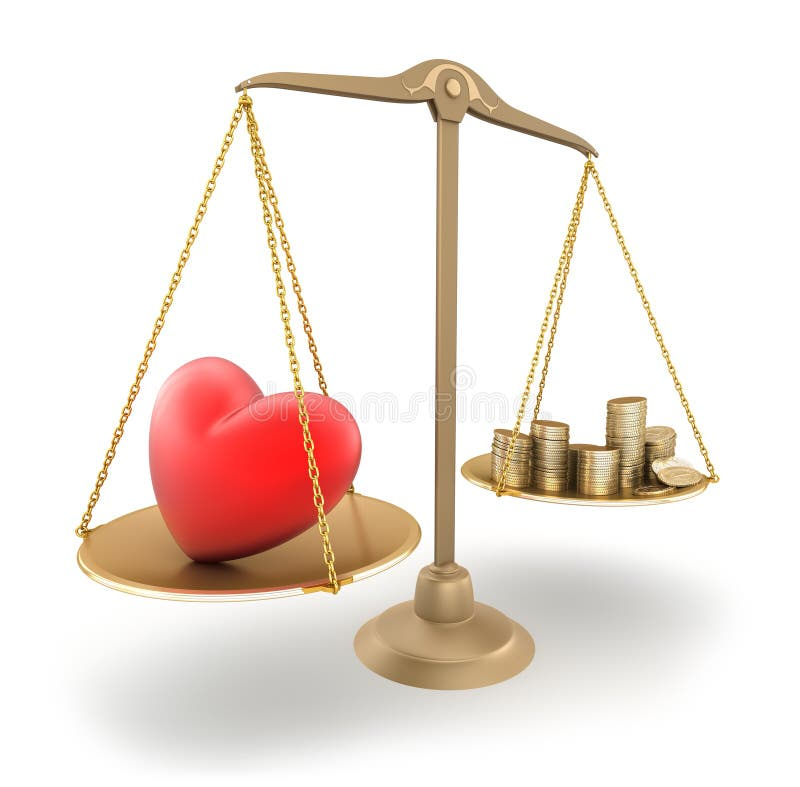The price of love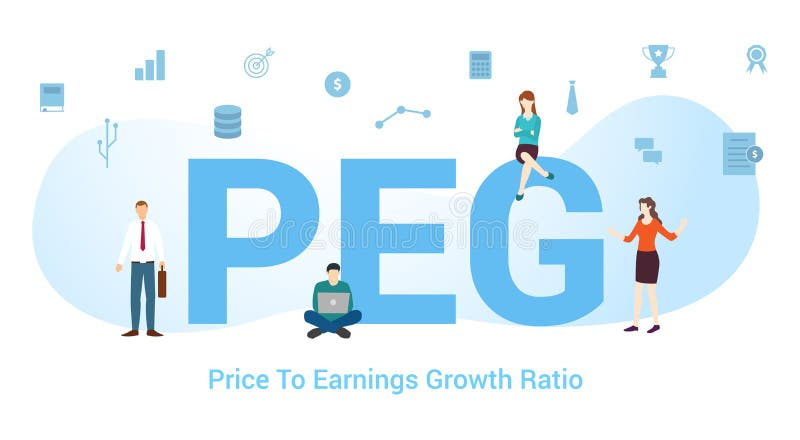Peg price to earnings growth ratio concept with big word or text and team people with modern flat style - vectorLibra, scale line icon, outline vector sign, linear style pictogram isolated on white. Price comparison symbol, logo illustration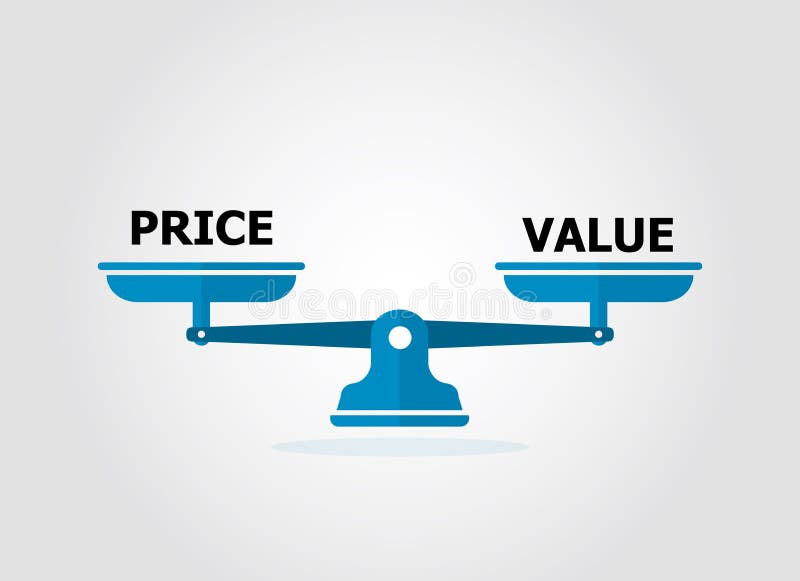Balance scale weight for price and value.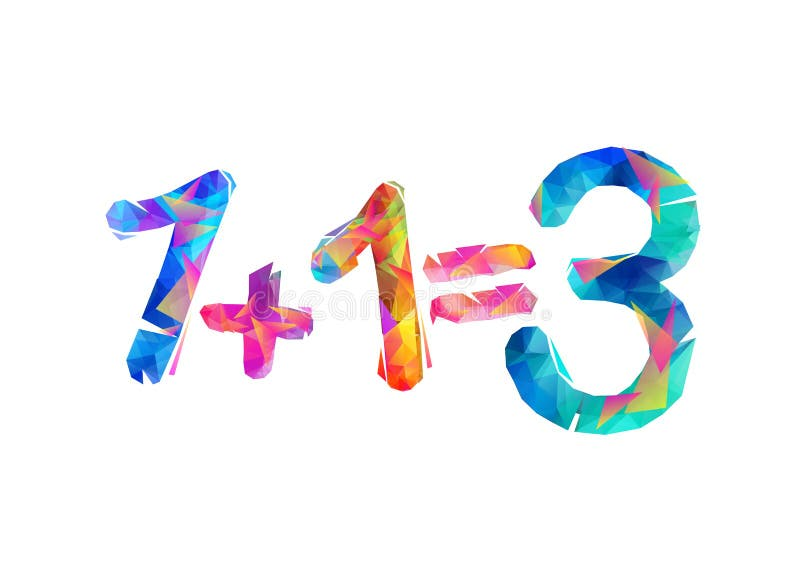One plus one equals three. Three for the price of twoidea lamp and money dollars on scales. vector modern symbol
More stock photos from Neeraj Charurvedi's portfolio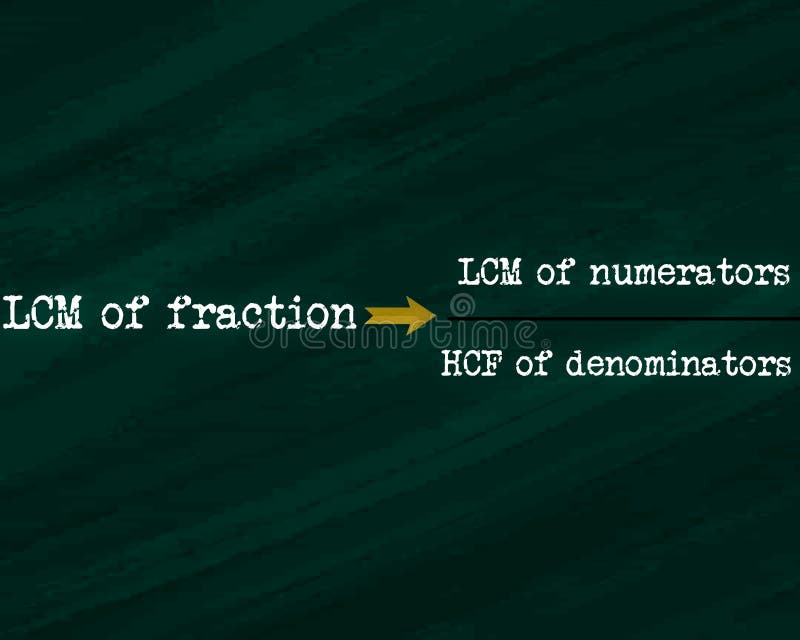lcm of fraction equal to LCM of numerator upon HCF of denominators equation displayed on chalkboard concept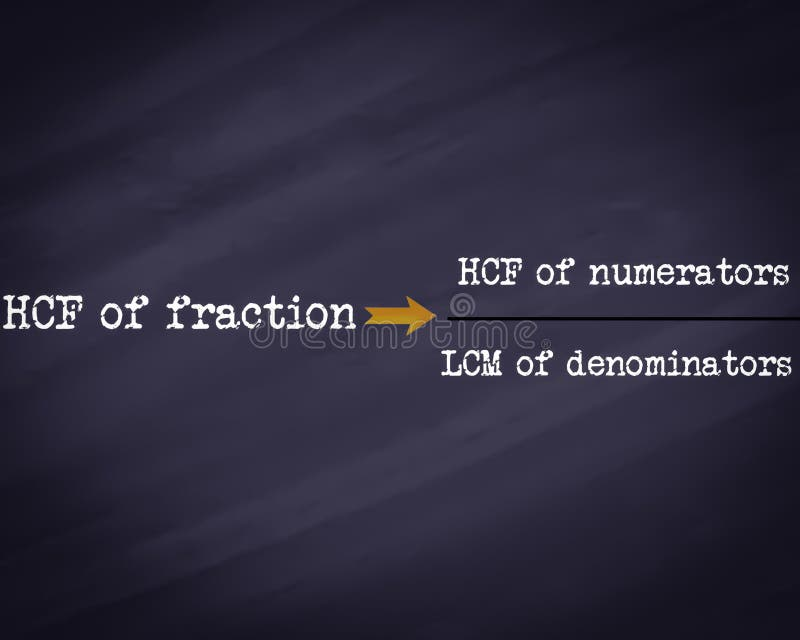HCF of fraction equal to HCF of numerators upon LCM of denominators equation displayed on chalkboard concept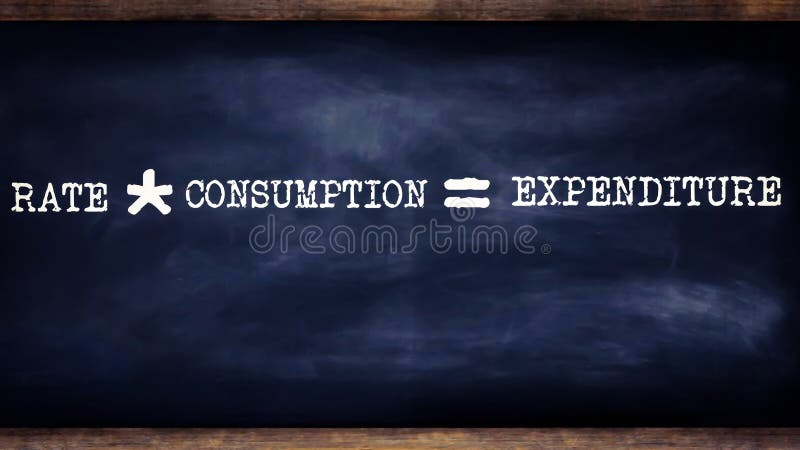rate into consumption equal to expenditure equation written on chalkboard concept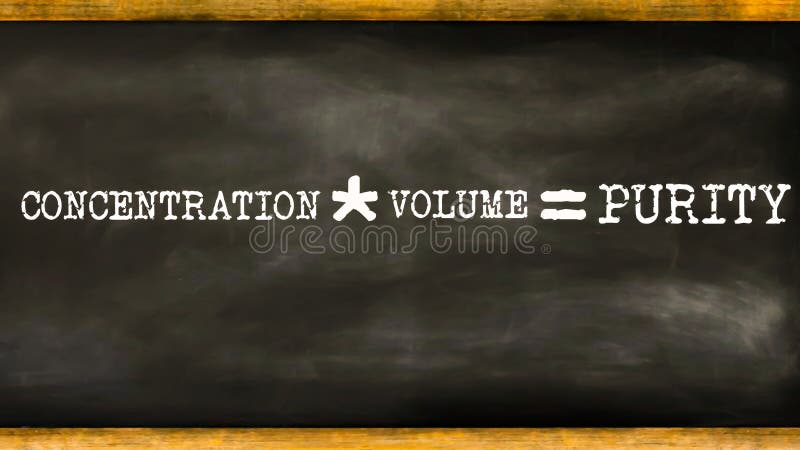concentration multiply by volume mathematical equation displayed on chalkboard concept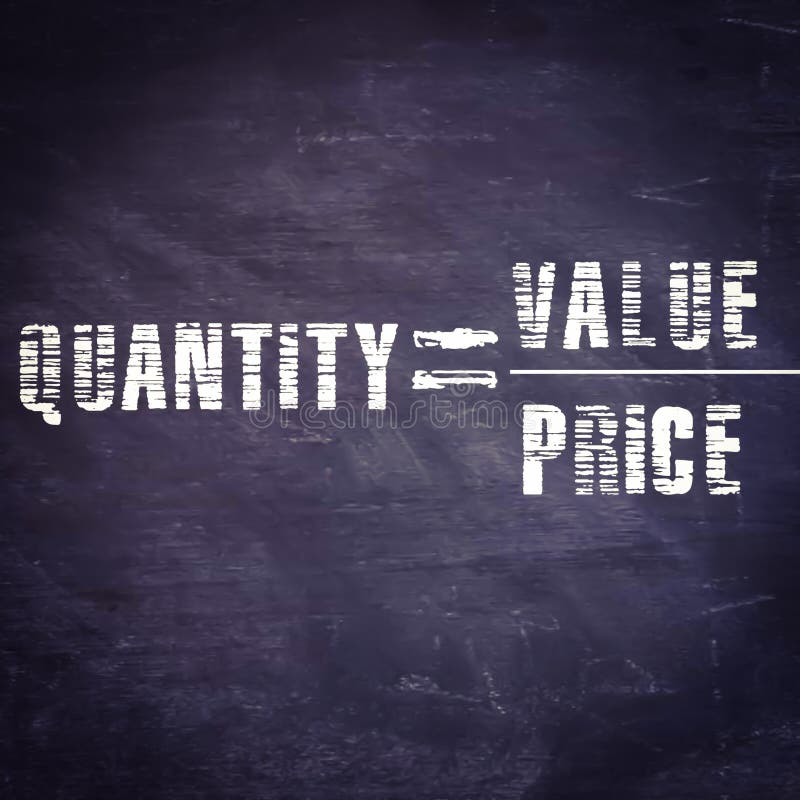quantity equal to value upon price equation displayed on chalkboard concept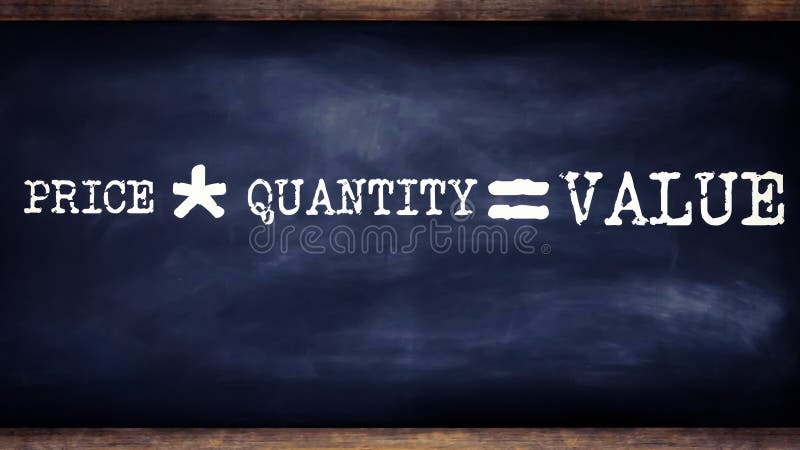price multiplication by quantity equal to value business related terminology displayed on chalkboard illustrations